Tamilnadu State Board New Syllabus Samacheer Kalvi 11th Maths Guide Pdf Chapter 4 Combinatorics and Mathematical Induction Ex 4.3 Text Book Back Questions and Answers, Notes.

## Tamilnadu Samacheer Kalvi 11th Maths Solutions Chapter 4 Combinatorics and Mathematical Induction Ex 4.3

Question 1.
If nC12 = nC9 find 21Cn
Given nC12 = nC9
We have nCx = nCy ⇒ x = y or x + y = n
nC12 = nC9
⇒ 12 + 9 = n ⇒ n = 21
⇒ 21Cn = 21C21 = 1Question 2.
If 15C2r – 1 = 15C2r + 4, find F.
Given 15C2r – 1 = 15C2r + 4
We have nCx = nCy ⇒ x = y or x + y = n
15C2r – 1 = 15C2r + 4
⇒ (2r – 1) + (2r + 4) = 15
4r + 3 = 15 ⇒ 4r = 12
r = $$\frac{12}{4}$$ = 3

Question 3.
If nPr = 720, If nCr = 120, find n, r = ?
Given nPr = 720, If nCr = 120r ! = 6
r ! = 3 × 2 × 1 = 3!
r = 3n (n – 1) (n – 2) = 720
n(n – 1) (n – 2) = 1o × 9 × 8
n = 10
∴ r = 3, n = 10Question 4.
Prove that 15C3 + 2 × 15C4 + 15C5 = 17C5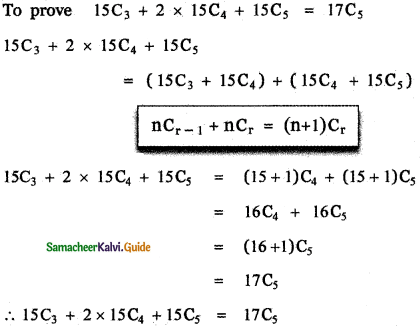Question 5.
Prove that 35C5 + $$\sum_{r=0}^{4}$$(39 – r) C4 = 40C5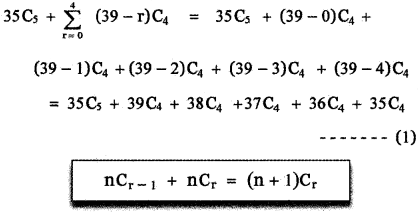Question 6.
If (n + 1)C8: (n – 3)P4 = 57 : 16, find n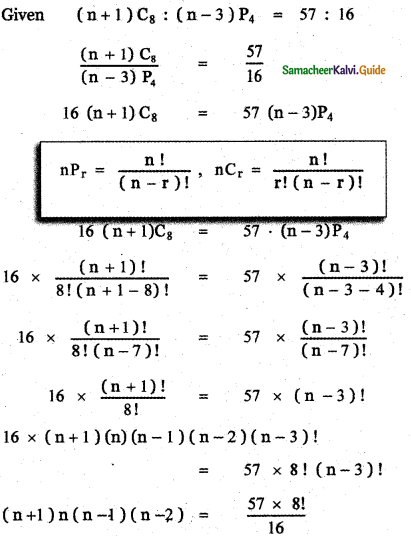(n + 1) n(n – 1)(n – 2) = 57 × 7 × 6 × 5 × 4 × 3
= 3 × 19 × 7 × 6 × 5 × 4 × 3
= (7 × 3) × (5 × 4)19 × (6 × 3)
= 21 × 20 × 19 × 18
= (20 + 1) × (20) × (20 – 1) × (20 – 2)
∴ n = 20Question 7.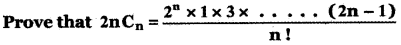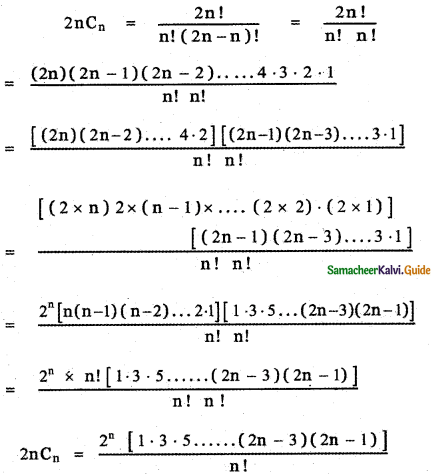Question 8.
prove that if 1 ≤ r ≤ n, then n × (n – 1)Cr – 1 = (n – r + 1) . nCr – 1Question 9.
(i) A kabaddi coach has 14 players ready to play. How many different teams of 7 players could the coach put on the court ?
Number of kabaddi players = 14
7 players must be selected from 14 players
Number of ways of selecting 7 players from 14 players is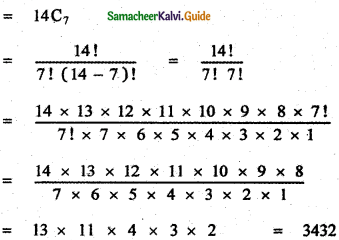(ii) There are 15 persons in a party and if each 2 of them shakes hands with each other, how many handshakes happen in the party?
Total number of person in the party = 15
Given if each 2 of the 15 persons shakes bands with each other.
∴ The total number of hand shakes is same as the number of ways of selecting 2 persons among 15 persons. This can be done in 15C2 ways.
Number of hand shakes = 15C2(iii) How many chords can be drawn through 20 points on a circle ?
Number given points on the circle = 20
A chord is obtained by joining any two points on the circle.‘Number of chords drawn through 20 points is same as the number of ways of selecting 2 points out of 20 points. This can be done 20C2 ways.
∴ The total number of chords = 20C2(iv) In a parking lot one hundred, one year old cars are parked. Out of them five are to be chosen at random for to cheek its pollution devices. How many different set of five cars can be chosen?
Number of cars in the parking lot = 100
Number of cars to be selected to check pollution device = 5
Number of ways of selecting 5 cars out of 100 cars(v) How many ways can a team of 3 boys, 2 girls and 1 transgender be selected from 5 boys, 4 girls and 2 transgenders ?
Number of boys = 5
Number of girls = 4
Number of transgender = 2
Number of ways of selecting 3 boys from 5 boys = 5c3
Number of ways of selecting 2 girls from 4 girls = 4C2
Number of ways of selecting one transgender from 2 transgenders = 2C1
Total number of ways of selection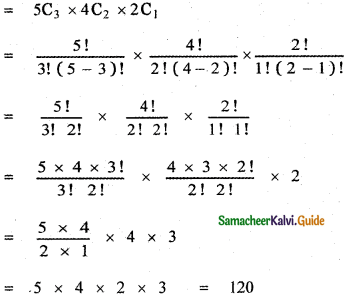Question 10.
Find the total number of subsets of a set with
(i) 4 elements
(ii) 5 elements
(iii) n elements.
(i) Subsets with 4 elements
Number of subsets with no element = 4Co
Number of subsets with one element = 4C1
Number of subsets with two elements = 4C2
Number of subsets with three elements = 4C3
Number of subsets with four elements = 4C4
∴ Total number of subsets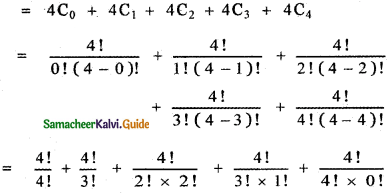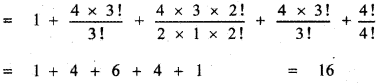(ii) Subsets with 5 elements:
Number of subsets with no element = 5C0
Number of subsets with one element = 5C1
Number of subsets with 2 elements = 5 C2
Number of subsets with 3 elements = 5C3
Number of subjects with 4 elements = 5C4
Number of subsets with 5 elements = 5C5
Total number of subjects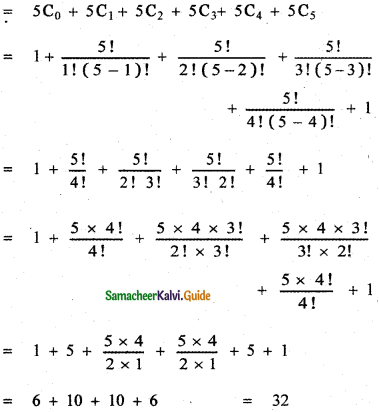(iii) Subsets with n elements
Number of subsets with no element = nC0
Number of subsets with 1 , 2 , 3, 4, …………. n elements are nC1, nC2, nC3, nC4 …………… nCnrespectively.
∴ Total number of subjects
= nC0 + nC1 + nC2 + nC3 + ………… + nCn
= Sum of the coefficients in the binomial expansion (x + a)n
= 2n

Question 11.
A Trust has 25 members.
(i) How many ways 3 officers can be selected?
(ii) In how many ways can a president, vice president and a secretary be selected?
Number of members in the trust = 25
(i) How many ways 3 officers can be selected?
The number of ways of selecting 3 officers from 25 members is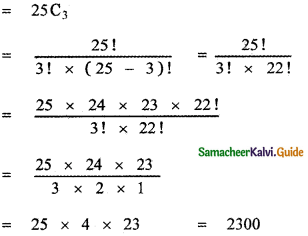(ii) In how many ways can a president, vice president, and secretary be selected?
From the 25 members, a president can be selected in 25 ways
After the president is selected, 24 persons are left out.
So a Vice President can be selected in (from 24 persons) 24 ways.
After the selection of Vice President, 23 persons are left out
So a secretary can be selected (from the remaining 23 persons) in 23 ways
So a president, Vice president, and a secretary can be selected in 25P3 ways
25P3 = 25 × 24 × 23 = 13800 waysQuestion 12.
How many ways a committee of six-person from 10 persons can be chosen along with a chairperson and a secretary?
Number of persons = 10
Number of committee members to be selected = 6
A committee must have a chairperson and a secretary, the remaining 4 members.
The number of ways of selecting a chairperson and a secretary from 10 persons is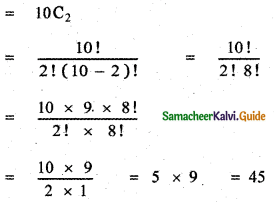After the selection of chairperson and secretary remaining number of persons = 8
Number of ways of selecting remaining 4 committee members from the remaining 8 persons= 2 × 7 × 5 = 70
∴ A number of ways of selecting the six committee members from 10 persons.
= 45 × 70 = 3150 waysQuestion 13.
How many different selections of 5 books can be made from 12 different books if,
(i) Two particular books are always selected?
Total number of books = 12
Number of books to be selected = 5
Given Two books are always selected.
Remaining number of books to be selected = 3
The number of ways of selecting the remaining 3 books from the remaining 10 books = 10C3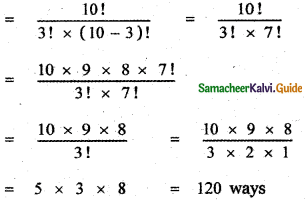(ii) Two particular books are never selected. Since two books are never selected, the total number of books is 10.
∴ The number of ways of selecting 5 books from 10 books= 2 × 9 × 2 × 7 = 252 ways

Question 14.
There are 5 teachers and 20 students. Out of them, a committee of 2 teachers and 3 students is to be formed. Find the number of ways in which this can be done. Further, find in how many of these committees
(i) a particular teacher is included?
(ii) a particular student is excluded?
The number of teachers = 5
Number of students = 20
The number of ways of selecting 2 teachers from 5 teachers is = 5C2 ways.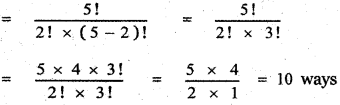The number of ways of selecting 3 students from 20 students is = 20C3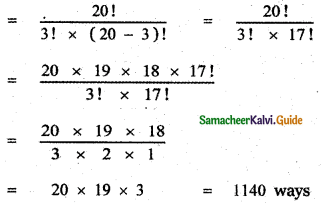= 20 × 19 × 3 = 1140 ways
∴ The total number of selection of the committees with 2 teachers and 5 students is = 10 × 1140 = 11400(i) A particular teacher is included.
Given a particular teacher is selected. Therefore, the remaining 1 teacher is selected from the remaining 4 teachers. Therefore, the number of ways of selecting 1 teacher from the remaining 4 teacher = 4C1 ways = 4 ways
The number of ways of selecting 3 students from 20 students = 20C3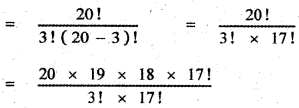Hence the required number of committees
= 1 × 4 × 1140 = 4560

(ii) A particular student is excluded The number of ways of selecting 2 teachers from 5 teachers is = 5C2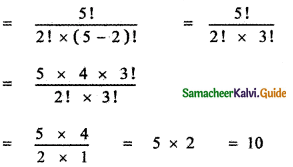A particular student is excluded
∴ The number of remaining students = 19
Number of ways of selecting 3 students from 19 students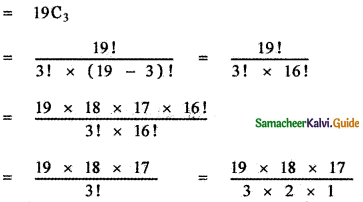= 19 × 3 × 17 = 969
∴ The required number of committees = 10 × 969 = 9690Question 15.
In an examination, a student has to answer 5 questions, out of 9 questions in which 2 are compulsory. In how many ways a student can answer the questions?
Total number of questions = 9
Number of questions to be answered = 5
Since 2 questions are compulsory, a student must select 3 questions from dying remaining 7 questions* The number of ways of selecting 3 questions from 7 questions is = 7C3
∴ The number of ways of answering 5 questions = 7C3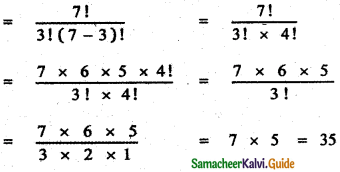Question 16.
Determine the number of 5 card combinations out of a check of 52 cards if there is exactly three aces in each combination.
Total number of cards in a pack 52
Number of aces = 4
Number of cards to be selected = 5
The number of ways of selecting 3 aces from 4 aces is 4C3
The number of ways of selecting the remaining 2 cards
from the remaining 48 cards (52 – 4 aces cards) = 48C2
∴ Required number of ways of selection
= 4C3 × 48C2
= 4C1 × 48C2= 4 × 24 × 47 = 4512

Question 17.
Find the number of ways of forming a committee of 5 members out of 7 Indians and 5 Americans, so that always Indians will be the majority in the committee.
Number of Indians = 7
Number of Americans = 5
Number of members in the committee = 5
Selection of 5 members committee with majority Indians

Case (i) 3 Indians and 2 Americans
The number of ways of selecting 3 Indians from 7 Indians is = 7C3
The number of ways of selecting 2 Americans from 5 Americans is = 5C2
Total number of ways in this case is = 7C3 × 5C2

Case (ii) 4 Indians and 1 American
The number of ways of selecting 4 Indians from 7 Indians is = 7C4
The number of ways of selecting I American from 5 Americans is = 5C1
The total number of ways, in this case, is = 7C4 × 5C1Case (iii) 5 Indians no American
Number of ways of selecting 5 Indians from
7lndiansis = 7C5
Total number of ways, in this case, = 7C5 × 5C0
∴ Total number of ways of forming the committee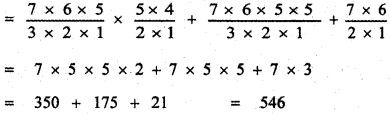= 7 × 5 × 5 × 24 – 7 × 5 × 5 + 7 × 3
= 350 + 175 + 21 = 546

Question 18.
A committee of 7 peoples has to be formed from 8 men and 4 women. In how many ways can this be done when the committee consists of
(i) Exactly 3 women?
(ii) Atleast 3 women?
(iii) Almost 3 women?
Number of men = 8
Number of women = 4
Number of peoples in the committee = 7

(i) Exactly 3 women
In a 7 member committee, women must be 3. Therefore, the remaining 4 must be men.
The number of ways of selecting 3 women from 4 women = 4C3
The number of ways of selecting 4 men from 8 men = 8C4
∴ The total number of ways of selection is = 4C3 × 8C4= 8 × 7 × 5 = 280(ii) At least 3 women
The 7 members committee must contain atleast 3 women
∴ We have the following possibilities
(i) 4 women + 3 men
(ii) 3 women + 4 men

case (i) 4 women + 3 men
The number ways of selecting 4 women .from
4 women is = 4C4 = 1 way
The number of ways of selecting 3 men from 8 men = 8C3∴ The total number of ways = 1 × 56 = 56

case (ii) 3 women + 4 men
The number of ways of selecting 3 women from 4 women is = 4C3
The number of ways of selecting 4 men from 8 men is = 8C4
∴ The total number of ways = 4C3 × 8C4= 8 × 7 × 5 = 280
∴ The required number of ways of forming the committee = 56 + 280 = 336

(iii) atmost 3 women
The 7 members must contain at most 3 women,
∴ we have the following possibilities
(i) No women + 7 men
(ii) 1 women + 6 men
(iii) 2 women + men
(iv) 3 women + 4 men

Case (i) 0 women + 7 men
The number of ways of selecting O women from 4 women is = 4C0
The number of ways of selecting 7 men from 8 men is = 8C7
Total number of ways = 4C0 × 8C7

Case (ii) 1 women + 6 men
The number of ways of selecting 1 woman from 4 women is = 4C1
The number of ways of selecting 6 men from 8 men is = 8C6
Total number of ways = 4C1 × 8C6

Case (iii) 2 women + 5 men
The number of ways of selecting 2 women from 4 women is = 4C3
The number of ways of selecting 4 men from 8 men is = 8C4
∴ Total number of ways = 4C3 × 8C4
∴ The required number of ways of forming the committeeQuestion 19.
7 relatives of a man comprise 4 ladies and 3 gentlemen, his wife also has 7 relatives: 3 of them are ladies and 4 gentlemen. In how many ways can they invite a dinner party of 3 ladies and 3 gentlemen so that there are 3 of the men’s relatives and 3 of the wife’s relatives?
Number of relatives of a man = 7
Number of relatives of the man’s wife = 7
The dinner party consists of 3 ladies and 3 gentlemen.
For the dinner party, 3 persons from the man’s relatives and 3 persons from the man’s wife’s relatives are invited.
Then we have the following possibilities for the different possible arrangements.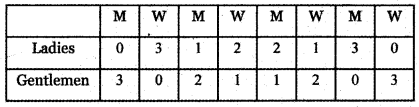Required number of ways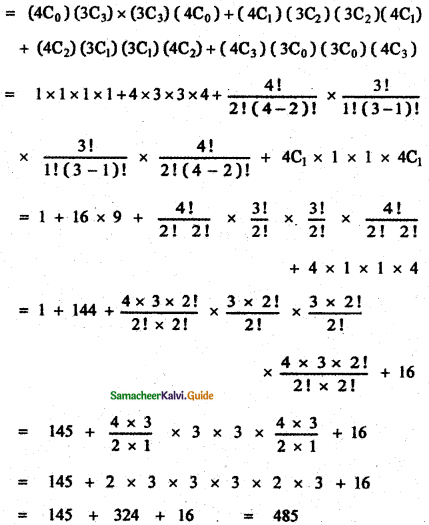Question 20.
A box contains two white balls, three black balls, and four red balls. In how many ways can three balls be drawn from the box, if atleast one black ball is to be included in the draw?
Number of white balls = 2
Number of black balls = 3
Number of red balls = 4
Number of balls drawn = 3 balls with atleast 1 blackball
We have the following possibilities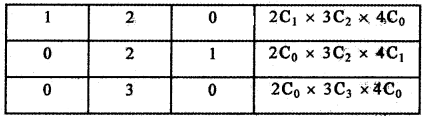Required number of ways of drawing 3 balls with atleast one black ball.Question 21.
Find the number of strings of 4 letters that can be formed with the letters of the word EXAMINATION.
There are 11 fetters in the word EXAMINATION,
They are AA, II, NN, E, X, M, T, O The four-letter strings may have
(i) 2 alike letters of one kind and 2 alike tetters of the second kind.
(ii) 2 alike fetters and 2 different letters.
(iii) All different fetters.

(i) 2 alike letter of one kind and 2 alike letters of the second kind:
There are three sets of 2 alike letters AA, II, NN. Out of these sets, two sets can be selected in 3C2 ways. So there are 3C2 groups each of which còntains 4 letter
strings out of which 2 are alike of one kind type and 2 are alike of the second type.
4 letters in each group can be arranged in $$\frac{4 !}{2 ! \times 2 !}$$ ways.
Hence the total number of strings consisting of two alike letters of one kind and 2 alike letters of the second kind(ii) 2 alike letter and 2 different letters:
Out of sets of two alike letters, one set can be chosen in 3C1 ways. From the remaining 7 distinct letters, 2 letters can be chosen in 7C2 ways. Thus 2 alike letters and 2 different letters can be selected in (3C1 × 7C2) ways. There are ( 3C1 × 7C2) groups of 4 letters each. Now letters of each group can be arranged among themselves in $$\frac{4 !}{2 !}$$ ways.

Hence the total number of strings consisting of 2 alike and 2 distinct letters,(iii) All different letters
There are 8 different letters
E, X, A, M, I, N, T, O

Out of which 4 can be selected in 8C4 ways. So there are 8C4 groups of 4 letters each The letter in each of 8C4 groups’ can be arranged in 4! ways.
∴ The total number of 4. letter strings in which all letters are distinct = 8C4 × 4!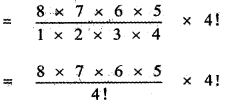= 56 × 30 = 1680 strings
Hence the total number of 4 letter strings
= 18 +756 + 1680
= 2454 stringsQuestion 22.
How many triangles can be formed by joining 15 points on the plane, in which no line joining any three points?
Number of points 15
To form a triangle we need 3 non – collinear points.
The number of ways of se1ecting 3 non-collinear points from 15 points is = 15C3Question 23.
How many triangles can be formed by 15 points of which 7 of them 11e on one line and the remaining 8 on another parallel ‘line?
To form a triangle we need 3 non-collinear points. Take the 7 points lying on one line be group A and the remaining 8 points lying on another parallel line be group B.
We have the following possibilities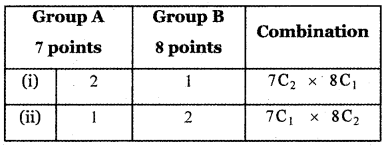∴ Required number of ways of forming the triangleQuestion 24.
There are 11 points in a plane. No three of these lie in the same straight line except 4 points which are collinear. Find
(i) The number of straight lines obtained from the pairs of these points?
(ii) The number of triangles that can be formed which the vertices as their points.
Number of points in a plane = 11
No three of these points lie in the same straight line except 4 points.

(i) The number of straight lines that can be obtained from the pairs of these points
Through any two points, we can draw a straight line.
∴The number straight lines through any two points of the given 11 points = 11C2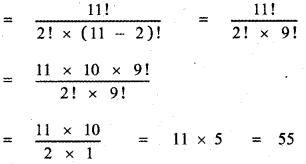Given that 4 points are collinear. The number of straight lines through any two points of these 4 points is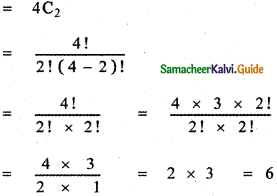Since these 4 points are collinear, these 4 points contribute only one line instead of the 6 lines.
The total number of straight lines that can be drawn through 11 points on a plane with 4 of the points being collinear is = 55 – 6 + 1 = 50

(ii) The number of triangles that can be formed for which the points are their vertices.
To form a triangle we need 3 non – collinear points. We have the following possibilities.

(a) If we take one point from 4 collinear points and 2 from the remaining 7 points and join them.

The number of ways of selecting one point from the 4 collinear points is = 4C1 ways
The number of ways of selecting 2 points from the remaining 7 points is = 7C2
The total number of triangles obtained in this case is = 4C1 × 7C2
∴ The total number of triangles obtained in this case is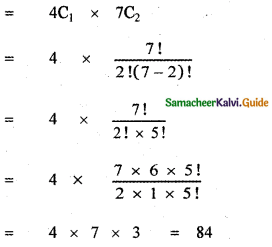(b) If we select two points from the 4 collinear points and one point from the remaining 7 points then the number of triangles formed is(c) If we select all the three points from the 7 points then the number of triangles formed is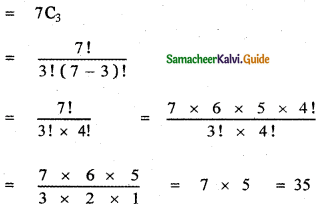∴ The total number of triangles formed are
= 84 + 42 + 35 = 161Question 25.
A polygon has 90 diagonals. Find the number of sides.
Let the number sides of the polygon be n.
The number of diagonals of a polygon with n sides = $$\frac{n(n-3)}{2}$$
Given $$\frac{n(n-3)}{2}$$ = 90
n2 – 3n = 180
n2 – 3n = 180
n2 – 3n – 180 = 0
n2 – 15n + 12n – 180 = 0
n (n – 15 ) + 12 (n – 15) = 0
(n + 12) (n – 15) = 0
n + 12 = 0 or n – 15 = 0
n = – 12 or n = 15
But n = – 12 is not possible
∴ n = 15
∴ The number of sides of the polygon having 90 diagonals is 15.